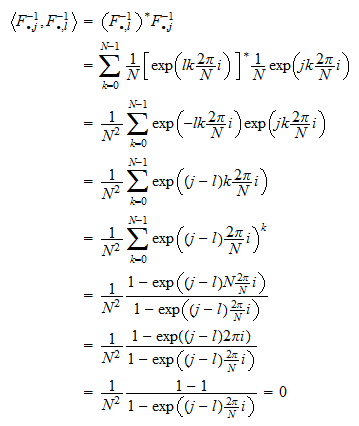StatLect

# Discrete Fourier transform

The Discrete Fourier Transform (DFT) is a linear operator used to perform a particularly useful change of basis.

It transforms a vector into a set of coordinates with respect to a basis whose vectors have two important characteristics:

1. they are orthogonal;

2. their entries are samples of the same periodic function taken at different frequencies.## Definition

Here is a definition.

Definition Letbe anvector. The discrete Fourier transform ofis anvectorwhose entries satisfywhereis the imaginary unit.

## Zero-based indexing

Zero-based indexing is often used to denote the entries of the vectorsand, as follows:In other words, the indices of the entries start from(not from, as it is customary for vectors) and are enclosed in square brackets.

## Periodicity

Remember thatSince the sine and the cosine are periodic functions with period, the functionis a periodic function ofwith period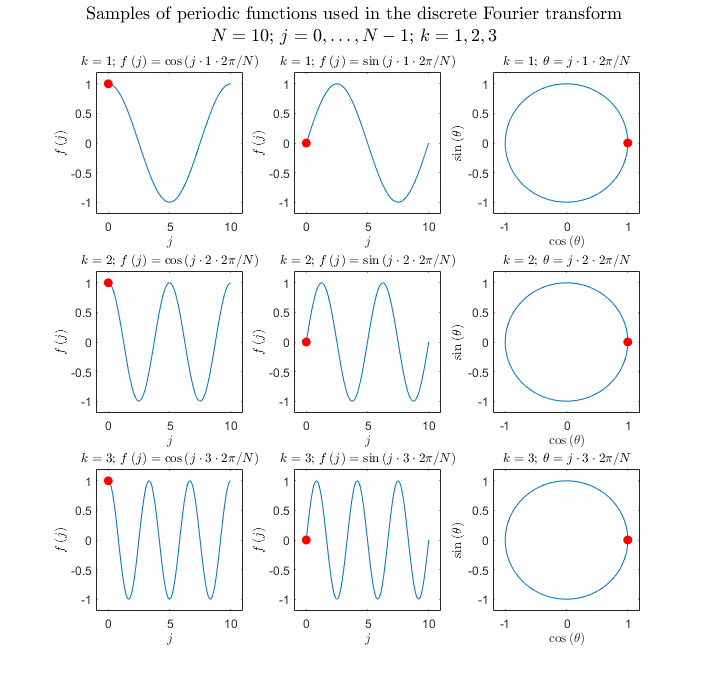## Inverse transform

The DFT is easily invertible.

Proposition Letandbe anvector and its discrete Fourier transform respectively. Then,Proof

We can write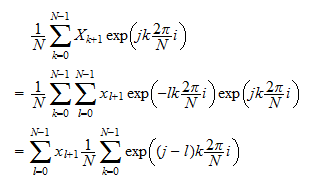If, thenIf, we can use the geometric series formulato writewhere in stepwe use the fact thatis a function ofthat has period. By putting together the equations above, we get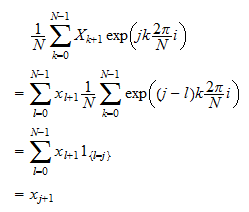## Fourier matrix

We can use a matrix to gather the values of the periodic functions used in the discrete Fourier transform.

Defineso thatThen, we can define theFourier matrix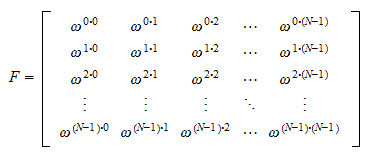We can useto write the DFT in matrix formBy using the definition of matrix multiplication, you can easily check that this equation is equivalent to the equations we have used above to define the DFT.

The equation makes clear that the DFT is a linear operator with matrix.

Note thatis a symmetric matrix.

## Inverse Fourier matrix

The inverse transform can be written asThe entries of the inverse Fourier matrixhave already been derived above: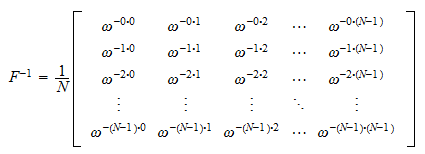## Frequency-domain representation

Denote bythe the-th column of.

Remember that post-multiplying a matrix by a column vector is the same as taking a linear combination of the columns of the matrix.

Therefore, we can write the inverse transform asThis is called frequency-domain representation of.

In other words,can be written as a linear combination of (samples of) periodic functionsthat have different frequencies.

The coefficients of the linear combination are.

## Orthogonality

The columns of the Fourier matrix and of its inverse are orthogonal to each other.

Proof

Consider two columns of the Fourier matrixand, with. Their inner product is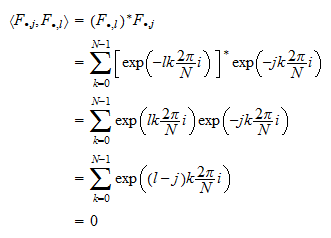wheredenotes conjugate transposition. The fact that the sum in the penultimate line is equal to zero was proved previously (see the the formula for the inverse transform). We leave as an exercise (see below) the proof that the columns of the inverse Fourier matrix are orthogonal.

However, the Fourier matrix, as defined above, is not unitary because its columns do not have unit norm.

Actually, all the columns have norm equal to.

Proof

Consider a column of the Fourier matrix. Its norm is: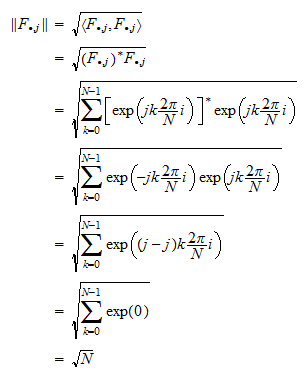To make the Fourier matrix unitary some authors define the Discrete Fourier Transform as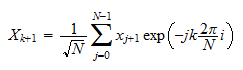in which case the inverse transform isThis is sometimes called the Unitary DFT.

The Fourier matrix of the Unitary DFT isIts inverse is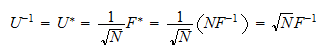wheredenotes conjugate transposition.

More details about the DFT can be found in the following lectures:

## Solved exercises

Below you can find some exercises with explained solutions.

### Exercise 1

Prove that the columns of the inverse Fourier matrix are orthogonal to each other.

Solution

Consider two columns of the inverse Fourier matrixand, with. Their inner product is